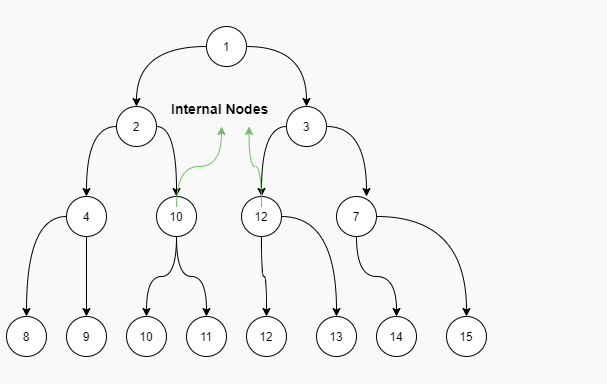Problem title
Difficulty
Avg time to solve

K Max Sum Combinations
Easy
10 mins
Maximum In Sliding Windows Of Size K
Moderate
20 mins
Generate Binary Numbers
Easy
10 mins
K subsets with equal sum
Easy
15 mins
Combination Sum
Easy
15 mins
Linear Probing
Easy
15 mins
Number of rectangles in a grid
Easy
15 mins
Closest Leaf To Given Node In Binary Tree
Moderate
15 mins
Max Stack
Easy
15 mins
Count triplets in a sorted doubly linked list whose sum is equal to a given value x
Moderate
15 mins10

# Except Boundary

Difficulty: MEDIUM
Avg. time to solve
36 min
Success Rate
65%

Problem Statement

#### Given a Binary Tree, find and return the sum of all the internal nodes. Internal nodes consist of all the nodes which are not at the boundary.

##### Example:
`````` Input:
````````````Output:

The internal Node is only 5, and the other nodes are boundary nodes.
Sum: 5
``````
##### Input Format:
``````The first line contains an Integer 'T' which denotes the number of test cases or queries to be run. Then the test cases follow.

The first line of input contains the elements of the tree in the level order form separated by a single space.

If any node does not have left or right child, take -1 in its place. Refer to the example below.

Example:

Elements are in the level order form. The input consists of values of nodes separated by a single space in a single line. In case a node is null, we take -1 in its place.

For example, the input for the tree depicted in the below image would be :
````````````1
2 3
4 -1 5 6
-1 7 -1 -1 -1 -1
-1 -1

Explanation :
Level 1 :
The root node of the tree is 1

Level 2 :
Left child of 1 = 2
Right child of 1 = 3

Level 3 :
Left child of 2 = 4
Right child of 2 = null (-1)
Left child of 3 = 5
Right child of 3 = 6

Level 4 :
Left child of 4 = null (-1)
Right child of 4 = 7
Left child of 5 = null (-1)
Right child of 5 = null (-1)
Left child of 6 = null (-1)
Right child of 6 = null (-1)

Level 5 :
Left child of 7 = null (-1)
Right child of 7 = null (-1)

The first not-null node (of the previous level) is treated as the parent of the first two nodes of the current level. The second not-null node (of the previous level) is treated as the parent node for the next two nodes of the current level and so on.

The input ends when all nodes at the last level are null (-1).
``````
##### Note :
``````The above format was just to provide clarity on how the input is formed for a given tree.

The sequence will be put together in a single line separated by a single space. Hence, for the above-depicted tree, the input will be given as:

1 2 3 4 -1 5 6 -1 7 -1 -1 -1 -1 -1 -1
``````
##### Output Format :
``````For each test case, return the sum of internal nodes.

Output for every test case will be denoted in a separate line.
``````
##### Note:
``````You do not need to print anything; It has already been taken care of.
``````
##### Constraint :
``````1 <= T <= 100
1 <= N <= 1000
-10^6 <= DATA <= 10^6

Time Limit : 1 sec
``````
##### Sample Input 1:
``````2
1 2 3 4 5 6 7 9 -1 -1 1 -1 1 -1 1 -1 -1 -1 -1 -1 -1 -1 -1
1 2 3 4 10 12 7 8 9 10 11 12 13 14 15  -1 -1 -1 -1 -1 -1 -1 -1 -1 -1 -1 -1 -1 -1 -1 -1
``````
##### Sample Output 1:
``````11
22
``````
##### Explanation of the Sample Input1:
``````Here we have 2 test cases.

Test Case 1: For the given tree, node 5 and 6 are not the boundary nodes, hence they are internal nodes of the binary tree. Therefore the sum of internal nodes is 11.
````````````Test Case 2: For the tree in the above image, nodes 10 and 12 consists of the internal node, other nodes represent the boundary of the binary tree. Hence the sum would be 10+12=22
``````Console